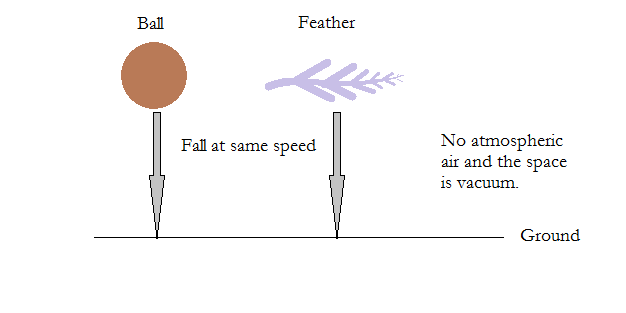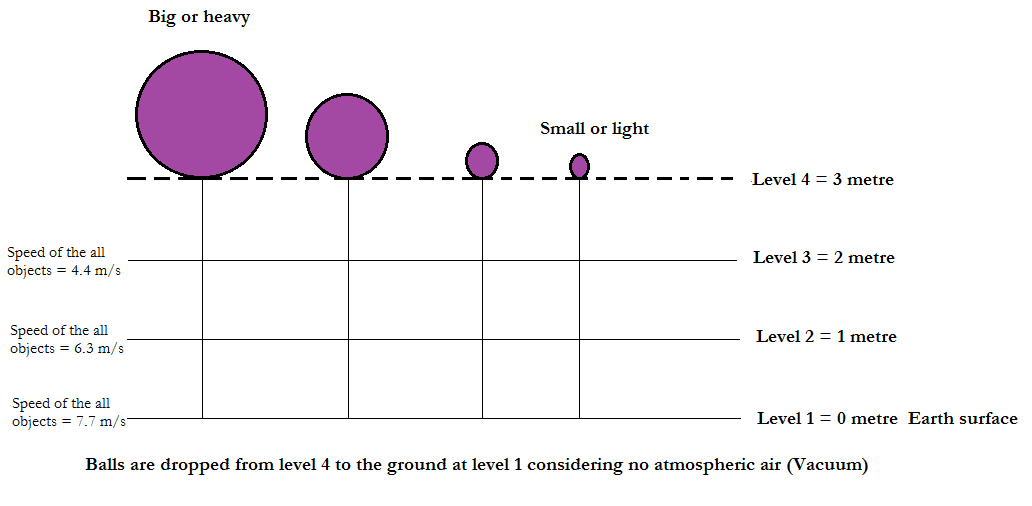# When you drop a heavy metal ball and a feather from a certain height which one does fall first?

## The speed of any objects which fall on the earth when it is simply dropped from a certain height is same without any medium (without atmospheric air). So the objects will fall to the ground at the same time. When you drop a heavy metal ball and a feather from a certain height the heavy metal ball and the feather will fall to the ground at the same time without atmospheric air (in vacuum).If there is no atmospheric air, then the heavy metal and the feather will fall at the same time.Earth has gravitational force. Due to this gravitational force every object on the earth falls to the ground if we drop it. If we through a ball upwards finally it falls to the ground. So earth is drawing objects towards its centre. But the speed at which the objects fall to the ground differs according to the height from which the objects fall.

Earth pulls the objects towards it only with respect to height not with respect to the mass of the objects.

If you accelerate the bike the speed of the bike increases. In the same way earth will accelerate an object at every metre at 9.81 m/s2. But the object may be small or big and heavy or light the acceleration is same. But there should not be atmospheric air. Because atmospheric air hindrance the objects due to friction. It changes the speed of the objects and changes their direction. For instance, it the feather is dropped it will fall long distance away from the dropped position due to the air movement.

See the figure below.Formula to find speed:

Velocity formula for an object is   u2+v2 = 2as.

Where,

u → initial velocity,

v →final velocity,

a → acceleration,

s → distance.

Here in our example we don’t have initial velocity. We simply drop the ball and the feather. So initial velocity, u = 0.

Acceleration is due to gravitational force, a = g = 9.81 m/s2.

Distance, s = 3 metre.

u2+v2 = 2as

02+v2 = 2x9.81x3

v2 = 58.86

v = √58.86

v = 7.67 m/s.

Speed of any object which falls from 3 metre height to the ground due to the gravitational force is 7.67 m/s without atmospheric air.

Summary:

The speed of any objects which fall on the earth when it is simply dropped from a certain height is same without any medium (without atmospheric air). So the objects will fall to the ground at the same time.

When you drop a heavy metal ball and a feather from a certain height the heavy metal ball and the feather will fall to the ground at the same time without atmospheric air (in vacuum).

#### What's Your Reaction?1100001